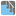• # Need some hint

## Question related to missionStair Steps

My logic is wrong because I tried to solve it in a sequential way. I need some hint on how to properly solve this.

Here's my code. It fails the last test because it always looks for "the coming 2 steps" without looking at the whole picture.

====

def checkio(numbers):

```reverse_order = list(reversed(numbers)) # reverse the order of numbers and assign it to new variable
ans = []

#shift through the last 2 list elements of the "reversed list"
while len(reverse_order)> 0:
l= reverse_order[-2:] # get the last 2 element
if l[-1] > 0:   # if the last element is positive, add it to the ans list
ans.append(l.pop())
reverse_order.pop() #remove this element

elif l[-1] < 0: # if the last element is negative, need to compare with the 2nd last element
if l[-2] > 0: #if the 2nd last element > 0, add this element to the ans list.
reverse_order.pop() #remove the last element
l.pop()
ans.append(l.pop()) #append and remove again
reverse_order.pop()

elif l[-2] <0:
if l[-2] > l[-1]:
reverse_order.pop()
l.pop()
ans.append(l.pop())
reverse_order.pop()

elif l[-2] < l[-1]:

ans.append(l.pop())
reverse_order.pop()

print('ans: ',ans)
print ('sum: ', sum(ans))

return(sum(ans))
```

# These "asserts" using only for self-checking and not necessary for auto-testing

if name == 'main': # assert checkio([5, -3, -1, 2]) == 6, 'Fifth' # assert checkio([5, 6, -10, -7, 4]) == 8, 'First' # assert checkio([-11, 69, 77, -51, 23, 67, 35, 27, -25, 95]) == 393, 'Second' assert checkio([-21, -23, -69, -67, 1, 41, 97, 49, 27]) == 125, 'Third' print('All ok')